# Test: Boundary Layer Theory - 2

## 10 Questions MCQ Test Topicwise Question Bank for Civil Engineering | Test: Boundary Layer Theory - 2

Description
Attempt Test: Boundary Layer Theory - 2 | 10 questions in 30 minutes | Mock test for Civil Engineering (CE) preparation | Free important questions MCQ to study Topicwise Question Bank for Civil Engineering for Civil Engineering (CE) Exam | Download free PDF with solutions
QUESTION: 1

### The maximum thickness of boundary layer in a pipe of radius r is

Solution:

The thickness of the boundary layer represented, by 8(greek 'delta’) is arbitrarily defined as that distance from the boundary surface in which the velocity reaches 99% of the velocity of the main stream, in a pipe the free stream velocity is at the centre of the pipe. Therefore maximum thickness of boundary layer is r.

QUESTION: 2

### The velocity profile for turbulent boundary layer is:

Solution:

Velocity profile follows 1/7th power law for turbulent boundary layer.

QUESTION: 3

### The drag force experienced by an object in a fluid stream is

Solution:
QUESTION: 4

The correct relationship among displacement thickness ‘d’ momentum thickness ‘m’ and energy thickness ‘e’ is

Solution:

Displacement thickness,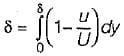Momentum thickness,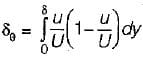Energy thickness,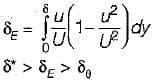QUESTION: 5

What is the momentum thickness for the boundary layer with velocity distribution u/U = y/δ ?

Solution:

The momentum thickness is given by,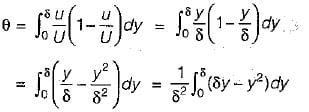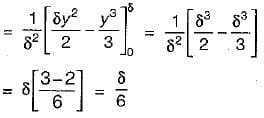QUESTION: 6

If x is the distance from leading edge, then the boundary layer thickness in laminar flow varies as

Solution:
QUESTION: 7

If δ1 and δ2 denote the boundary layer thicknesses at a point distance x from the leading edge on a fiat plate when the Reynolds numbers are 100 and 256 respectively, then the ratio of δ1 to δ2 will be

Solution: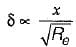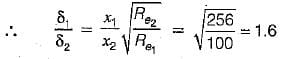QUESTION: 8

If δ1 is the thickness of laminar boundary layer at a distance x1 from the leading edge of the flat plate, the thickness δ2 at x2 = 2x1 is equal to

Solution:

For laminar boundary layer,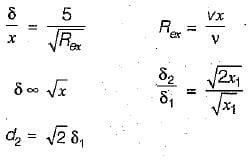QUESTION: 9

The displacement thickness δ* of a boundary layer is defined as δ* =

Solution:

Displacement thickness,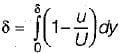Momentum thickness,,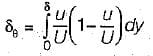Energy thickness,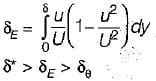QUESTION: 10

Separation of boundary layer takes place under

Solution:Use Code STAYHOME200 and get INR 200 additional OFF Use Coupon Code